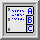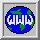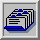#PMT gain vs. high voltage relation

The relation between the high voltage (HT) and the gain of the photomultipliers was determined from a set of dedicated "Laser Runs" with varying high voltages in Februar (runs 5012-5026).

The gain, defined as normalized laser amplitude, versus high voltage relation was fitted with a power law. The results of such a linear fit to ln(GAIN) vs ln[HT(Volts)] for one (the best..) module look like

Red lines denote the high voltages and corresponding (normalized) gains in September (higher HT) and June (lower HT). For forward rings the gains in June were up to 4 times smaller than in September (large extrapolation needed).

All gain fit values are stored in a single file with the format

```MODULE  SEP/JUN  sigma      power      sigma   HT_SEP  HT_JUN
```
which reads like
```CSI_0101_R  1.8108  0.0034    5.891185  0.018580   1043    943
CSI_0102_R  1.8191  0.0036    5.792681  0.019037   1019    919
CSI_0103_R  1.7199  0.0029    5.721974  0.017565   1106   1006
CSI_0104_R  1.7217  0.0019    5.608264  0.011676   1083    983
```

Here, the 1-st column is the module in question, 2-nd the calculated ratio of "SEP/JUN" gains, 3-rd its sigma, 4-th the power from the power law fit, 5-th column gives the standard deviation of "power", and 6-th and 7-th the HT in September and in June respectively (in Volts).

Usage of this file in general is straightforward. The ratio of 2 gains (G1/G2) for a given module knowing the corresponding high voltages (HT1 and HT2) is just:

G1/G2 = (HT1 /HT2)power

It may be interesting to notice that tubes with 8 dynodes have the powers around 6 and the tubes with 10 dynodes have the powers around 8.

##Web access

The data is available under gain_fits.dat.

##GSI local access

The file is accessible from the Linux system under
```    /d/kp3/bck/s185/pmt_gain_vs_hv/gain_fits.dat
```

Data by J. Lukasik, Web by Walter F.J. Müller
Last modified: Mon Oct 18 19:02:39 CEST 1999